Industry Article

# Resolving the Signal: Introduction to Noise in Delta-Sigma ADCs Part 2

February 13, 2018 by Bryan Lizon, Texas Instruments

## In part 2 of this series, I'll continue the fundamental ADC noise discussion by covering how to measure ADC noise, noise specifications in ADC data sheets, and absolute versus relative noise parameters.

This 12-part article series focuses on the impact of noise in delta-sigma ADCs. In Part 2, we continue the fundamental ADC noise discussion by covering how to measure ADC noise, noise specifications in ADC data sheets, and absolute versus relative noise parameters.

Part 1 of this series discusses noise in electrical systems, the causes of noise in a typical signal chain, inherent analog-to-digital converter (ADC) noise and the difference between noise in high- and low-resolution ADCs and can be found here

Before I explain how we measure ADC noise at Texas Instruments, it’s important to understand that when you look at ADC data sheet specifications, the goal is to characterize the ADC, not the system. As a result, the way TI tests ADC noise and the test system itself should demonstrate the capabilities of the ADC, not the limitations of the testing system. Therefore, using the ADC in a different system or under different conditions may lead to noise performance that varies from what is reported in the datasheet.

There are two methods by which we measure ADC noise. In the first method, we short the ADC’s inputs together to measure the slight variations in output code as a result of thermal noise. The second method involves inputting a sine wave with a specific amplitude and frequency (such as 1VPP at 1kHz) and reporting how the ADC quantizes the sine wave. Figure 1 demonstrates these types of noise measurements.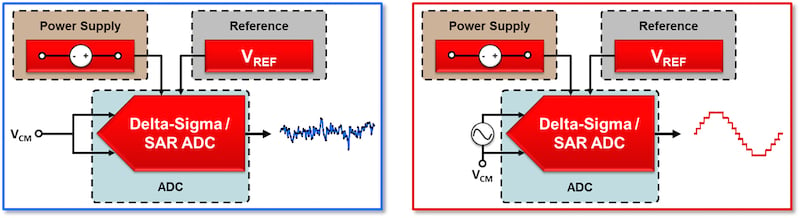##### Figure 1. Input-short test setup (a); sine-wave-input test setup (b)

Typically, we choose an individual ADC’s noise measurement method based on its target end application(s). For example, delta-sigma ADCs that measure slow-moving signals such as temperature or weight use the input-short test, which precisely measures performance at DC. Delta-sigma ADCs used in high-speed data-acquisition systems generally rely on the sine-wave-input method, where AC performance is critical. For many ADCs, the datasheet specifies both types of measurements.

For example, the 24-bit ADS127L01 from TI has a high maximum sampling rate of 512kSPS and a low passband-ripple wideband filter, which enable high-resolution AC signal sampling for test and measurement equipment. However, these applications often require accurate measurement of the signal’s DC component as well. As a result, we characterize not only the ADC’s performance with a range of AC input signals at multiple sample rates but also the ADS127L01’s DC performance using the input-short test.

### Noise Specifications in ADC Data Sheets

If you look at the ADS127L01’s data sheet – or any ADC data sheet, for that matter – you’ll see noise performance reported in two forms: graphically and numerically. Figure 2 shows a fast Fourier transform (FFT) of the ADS127L01’s noise performance using an input sine wave with an amplitude of -0.5dbFS and 4kHz frequency. From this plot, we calculate and report important AC parameters such as the signal-to-noise ratio (SNR), total harmonic distortion (THD), signal-to-noise ratio and distortion (SINAD) and effective number of bits (ENOB).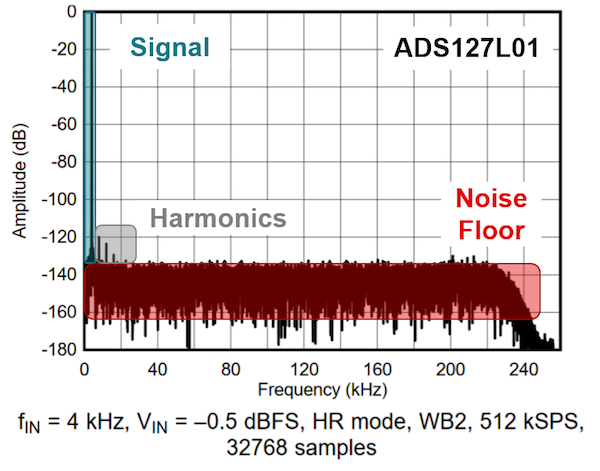##### Figure 2. Example ADS127L01 FFT with a 4kHz, -0.5dBFS input signal

For DC performance, a noise histogram shows the distribution of output codes for a specific gain setting, filter type and sample rate. From this plot, we calculate and report important DC noise performance parameters such as input-referred noise, effective resolution and noise-free resolution. (Note: many engineers use the terms “ENOB” and “effective resolution” synonymously to describe an ADC’s DC performance. However, ENOB is purely a dynamic performance specification derived from SINAD and is not meant to convey DC performance. Throughout the rest of this article series, I will use these terms accordingly. For more comprehensive parameter definitions and equations, see Table 1.)

Figure 3 shows the noise histogram for the ADS127L01.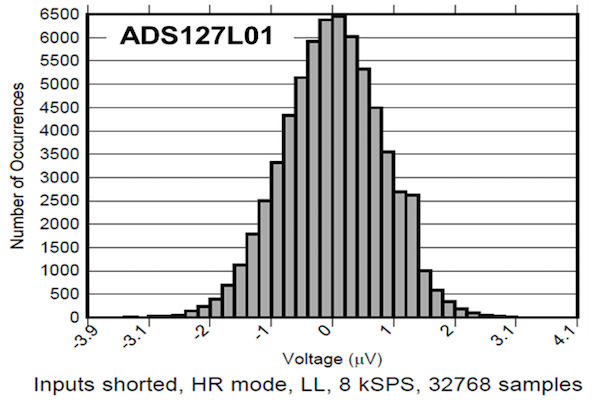##### Figure 3. Example ADS127L01 noise histogram

Like the FFT plot, the noise histogram provides important graphical information about DC noise performance. Since the noise histogram has a Gaussian distribution, the definition of average (root mean square [RMS]) noise performance is typically one standard deviation – the red-shaded region in Figure 4a.

In Figure 4b, the blue-shaded region depicts the peak-to-peak (VN,PP) noise performance of the ADC. Peak-to-peak noise is given as 6 or 6.6 standard deviations due to the crest factor of Gaussian noise, which is the ratio of the peak value to the average value. Peak-to-peak noise defines the statistical probability that the measured noise will be within this range. If your input signal also falls in this range, there is a chance that it will be obscured by the noise floor, resulting in code flicker. Additional oversampling will help reduce peak-to-peak noise at the cost of longer sampling time.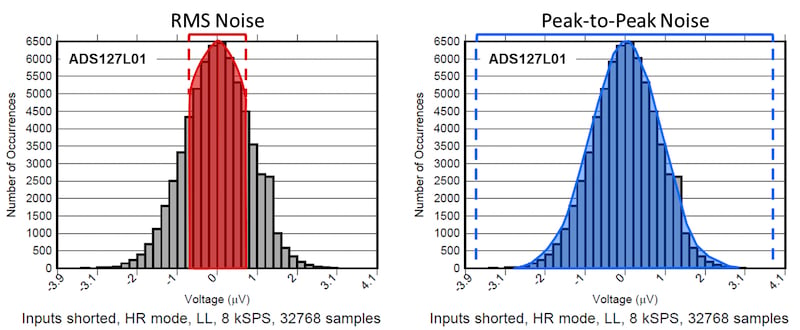##### Figure 4. ADS127L01 RMS noise (a); peak-to-peak noise (b)

You’ll also find the aforementioned AC and DC specifications numerically in the electrical characteristics section of any ADC data sheet. An exception to this rule involves ADCs with integrated amplifiers, where noise performance varies with gain as well as data rate. In such instances, there is generally a separate noise table for parameters such as input-referred noise (RMS or peak-to-peak), effective resolution, noise-free resolution, ENOB and SNR.

Table 1 summarizes AC and DC noise parameters, their definitions and equations.

Table 1: Typical ADC noise parameters with definitions and equations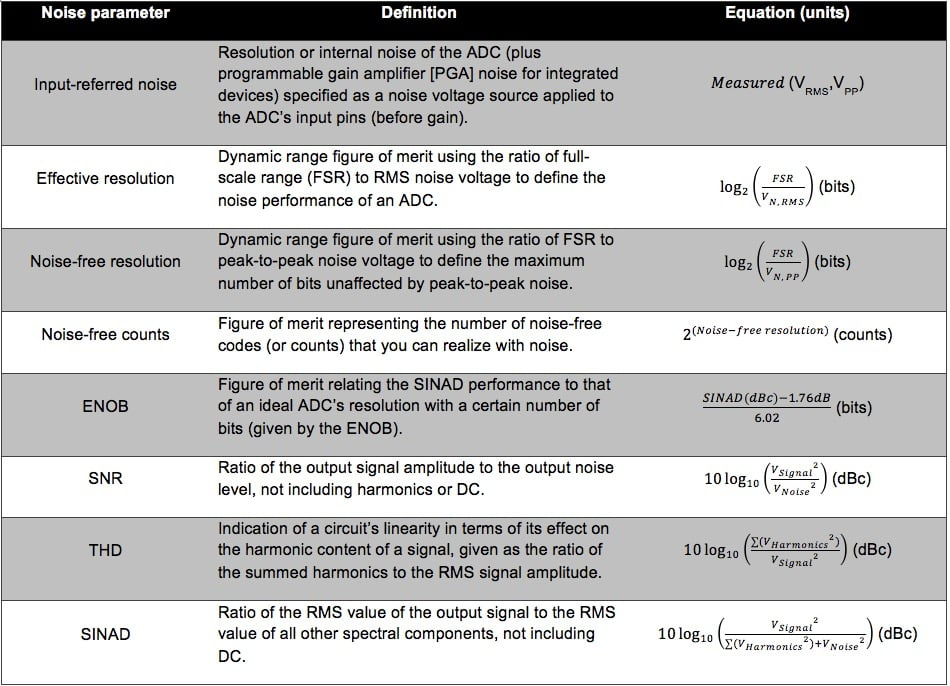### Absolute vs. Relative Noise Parameters

An important characteristic of all of the equations in Table 1 is that they involve some ratio of values. We define these as “relative parameters.” As the name implies, these parameters provide a noise performance metric relative to some absolute value, usually the input signal (decibels relative to carrier [dBc]) or the full-scale range (decibels relative to full scale [dBFS]).

Figure 5 shows an output spectrum of the ADS127L01 using an input signal at -0.5dBFS, where full scale is 2.5V. If you choose a system input signal that is not referenced to the same full-scale voltage, or if the input signal amplitude varies from the value defined in the data sheet, you should not necessarily expect to achieve data-sheet performance, even if all of your other input conditions are identical.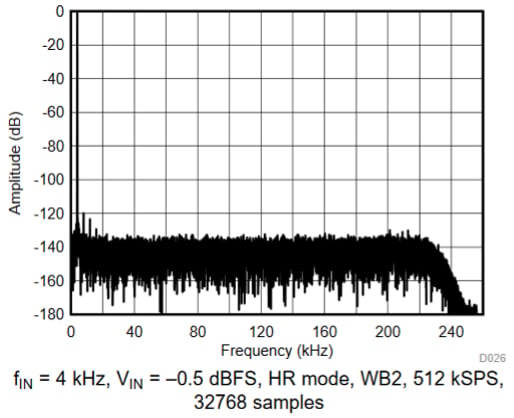##### Figure 5. ADS127L01 FFT with input voltage (VIN) referenced to full scale

Similarly, for DC noise parameters, you can see from Table 1 that effective resolution is relative to the ADC’s input-referred noise performance at the given operating conditions as well as at the ADC’s FSR. Since FSR depends on the ADC’s reference voltage, using a reference voltage other than what’s used in the datasheet has an effect on your ADC’s performance metrics.

For high-resolution ADCs, increasing the reference voltage increases the maximum input dynamic range, while input-referred noise stays the same. That is because high-resolution ADC noise performance is largely independent of the reference voltage. For low-resolution ADCs, where noise is dominated by least significant bit (LSB) size, increasing the reference voltage actually increases input-referred noise, while the maximum input dynamic range remains approximately the same. Table 2 summarizes these effects.

Table 2: Effect of changing reference voltage on ADC noise parameters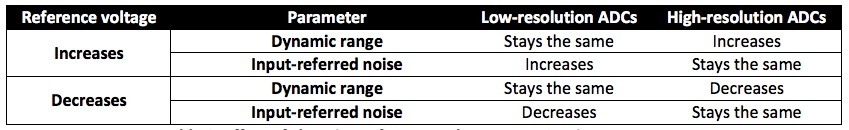Therefore, to characterize an ADC’s maximum dynamic range, most ADC manufacturers specify the effective resolution and noise-free resolution using the assumption that the FSR is maximized. Or, in other words, if your system does not use the maximum FSR (or whatever FSR the manufacturer used to characterize the ADC), you should not expect to achieve the effective or noise-free resolution values specified in the datasheet.

Let’s illustrate this point by using a 1V reference voltage with an ADC whose data-sheet noise is characterized by a reference voltage of 2.5V. Continuing with the ADS127L01 as an example, Figure 6 shows that using a 2.5V reference voltage and a 2kSPS date rate in very low power (VLP) mode results in 1.34µVRMS of input-referred noise and an effective resolution of 21.83 bits.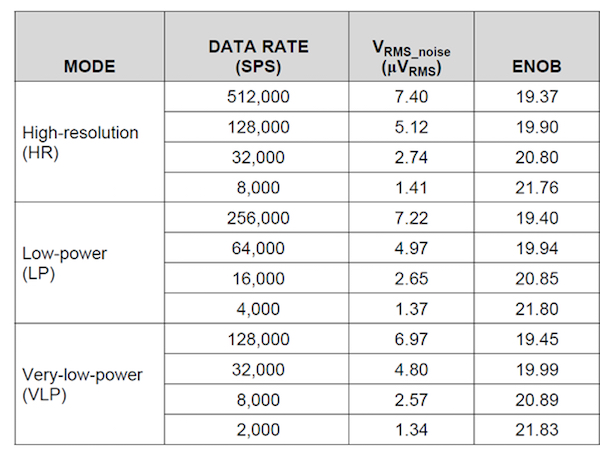##### Figure 6. ADS127L01 noise performance: low-latency filter, AVDD = 3V, DVDD = 1.8V and VREF = 2.5V

However, using a 1V reference voltage reduces the FSR to 2V. You can use this value to calculate the new expected effective resolution (dynamic range), given by Equation 1: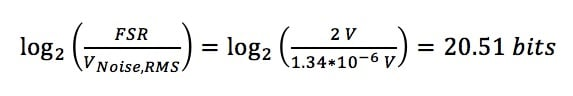Changing the reference voltage reduced the ADC’s FSR, which in turn reduced its effective resolution (dynamic range) compared to the datasheet value by more than 1.3 bits. Equation 2 generalizes this loss of resolution: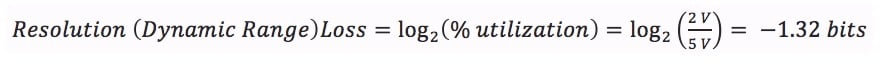where % utilization is simply the ratio of the actual FSR to the FSR at which the ADC’s noise is characterized.

While this apparent resolution loss may seem like a drawback to using high-resolution delta-sigma ADCs, recall that while the FSR is decreasing, the input-referred noise is not. Therefore, I suggest performing ADC noise analysis using an absolute noise parameter, or one that is measured directly. Using an absolute noise parameter eliminates the dependence on input signal and reference voltage characteristic of relative noise parameters. Additionally, absolute parameters simplify the relationship between ADC noise and system noise.

For ADC noise analysis, I recommend using input-referred noise. I’ve bolded this phrase because it’s not common practice to use input-referred noise to define ADC performance. In fact, a majority of engineers speak exclusively in terms of relative parameters such as effective and noise-free resolution and are deeply concerned when they cannot maximize those values. After all, if you need to use a 24-bit ADC to achieve 16-bit effective resolution, it feels like you’re paying for performance that the ADC cannot actually provide.

However, an effective resolution of 16 bits doesn’t necessarily tell you anything about how much of the FSR you use. You may only need 16 bits of effective resolution, but if the minimum input signal is 50nV, you will never be able to resolve that with a 16-bit ADC. Therefore, the true benefit of a high-resolution delta-sigma ADC is the low levels of input-referred noise it offers. It does not mean that effective resolution is unimportant – just that it is not the best way to parameterize a system.

Ultimately, if the ADC cannot resolve both the minimum and maximum input signals, maximizing SNR or effective resolution is irrelevant. And unlike effective resolution, you can typically derive the ADC’s required input-referred noise directly and easily from the system specifications. This characteristic makes input-referred noise analysis more flexible to system changes. Additionally, it enables easy comparison between different ADCs in order to choose a specific ADC for any application.

In the third installment of this article series, I’ll examine a resistive bridge design example in detail, using both relative and absolute noise parameters to define the system resolution to demonstrate the effectiveness of each. I’ll also show how each parameter type impacts ADC comparison and selection.

### Key Takeaways

Here is a summary of important points to help better understand noise in delta-sigma ADCs:

• Different measurements quantify different types of noise:
• To measure AC noise performance, use the AC-signal applied test.
• To measure DC noise performance, use the input-short test.
• ADC end applications typically determine the noise measurement type.
• Effective/noise-free resolution metrics? In general, assume that the input signal = FSR.
• There are two types of noise parameters:
• Relative – calculated using a ratio of measured values.
• Absolute – measured directly.
• Input-referred noise is the absolute measure of an ADC’s resolution (the smallest measurable signal). Noise-free-bits and effective resolution are relative parameters that describe the dynamic range of the ADC.

Industry Articles are a form of content that allows industry partners to share useful news, messages, and technology with All About Circuits readers in a way editorial content is not well suited to. All Industry Articles are subject to strict editorial guidelines with the intention of offering readers useful news, technical expertise, or stories. The viewpoints and opinions expressed in Industry Articles are those of the partner and not necessarily those of All About Circuits or its writers.